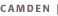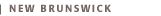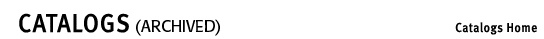# Courses (Statistics 960)50:960:183 Elementary Applied Statistics (R) (3) Frequency distribution, graphical representations, measures of central tendency and variability, elements of probability, the normal curve and its applications, sample versus population, estimating and testing hypotheses, regression and correlation analysis, nonparametric tests. Emphasis on applications. No prerequisite beyond the usual three years of high school mathematics. Credit will not be given for both this course and 50:830:215. 50:960:283 Introduction to Statistics I (R) (3) Elementary course in the principles and methods of statistics. Topics include measures of central tendency and dispersion, probability theory, random variables and probability distribution, binomial and normal distributions, central limit theorem, confidence intervals, and testing of hypotheses on mean(s) and proportion(s). Prerequisite: 50:640:121 or 130. Intended primarily for business majors and information systems/computer science majors. 50:960:284 Introduction to Statistics II (R) (3) A second introductory statistics course. Emphasizes the application of statistical techniques to data analysis. Topics include analysis of variance, nonparametric statistics, simple linear regression, correlation, multiple regression, time series, and index numbers. Prerequisite: 50:960:283. Intended primarily for business majors and information systems/computer science majors. 50:960:336 Applied Statistics (3) Descriptive statistics, probability, random variables, probability distributions, estimation and tests of hypotheses, and regression and correlation analysis. Emphasis on applications of these techniques to problems in the biological, physical, and social sciences. Prerequisite: 50:640:122. Intended primarily for applied mathematics majors but open to all qualified students. 50:960:337 Managerial Statistics (Intermediate) (3) An intermediate course oriented to business and managerial decisions and research in social sciences. Statistical decision making, a priori and a posteriori probabilities, quality control sampling, power curve solutions, sequential decisions, and research design. Design of sample surveys and study of replicated sampling plans. Prerequisite: 50:960:283 or permission of instructor. 50:960:340 Special Topics in Statistics (3) Aimed at students with any major who want to go beyond the first two statistics courses. Instructor provides proper description. Prerequisite: 50:960:284. 50:960:384 Statistical Data Analysis (3) Aimed at students who want to go beyond the first two statistics courses. Application of statistical techniques to analyze data. Topics include correlation and regression analysis, regression diagnostics, model building, design of experiments, and categorical data analysis. Use of computer packages for visual analysis and interpretation of data. Prerequisite: 50:960:284. 50:960:390 Introductory Computing for Statistics (3) Aimed at students who want to learn statistical computing along with or after the second statistics course. Introduces statistical computing using packages (Excel, SAS, etc.). Includes computing basic univariate statistics, generating random numbers, computing point estimates and confidence interval, testing of hypothesis, basic ANOVA, and regression. Pre- and corequisites: 50:960:283, 284. 50:960:452 Introduction to Biostatistics (3) Introduction to the principles and methods of statistical inference for advanced undergraduate and graduate students in the biological sciences. Topics include discussion of random variables, probability distributions, population, sample, measures of central tendency and dispersion, point and interval estimation, testing hypothesis, two-sample comparison, analysis of variance, linear regression and correlation model, and nonparametric methods. Emphasizes applications of statistical principles and analyses for biological sciences. Prerequisite: 50:640:121 or 130. 50:960:467 Introduction to Applied Multivariate Analysis (3) Aimed at students with any major who want to go beyond the first two statistics courses. Introduction to applied multivariate analysis through multivariate normal distribution. Topics include comparison of mean vector, multiple linear regression, discriminant analysis, principal components, factor analysis, and other applied multivariate topics. Use of statistical packages to perform all the multivariate computation and its interpretation. Prerequisite: 50:960:284. 50:960:476 Introduction to Sampling (3) Application of the principles of sampling to economic procurement or assessment of data. Introduction to various sampling procedures. Emphasis on the design and control phases of investigation. Applications of the techniques to large-scale surveys, accounting and auditing, and operations research. Prerequisite: 50:960:283 or 336 or permission of instructor. 50:960:481,482 Mathematical Theory of Statistics (3,3) First semester: theory of probability, discrete and continuous probability distributions, introduction to statistical inference. Second semester: further study of distribution functions, correlation and regression, analysis of variance and design of experiments, nonparametric methods, sequential sampling. Prerequisite: First course in calculus or permission of instructor. 50:960:483 Statistical Quality Control (3) Basic course in modern statistical quality control. Examines statistical measures, histogram analysis, construction and analysis of control charts for variables and attributes, use of Dodge-Roming and military standards acceptance sampling plans, and statistical aspects of tolerances. Prerequisite: 50:960:283 or permission of instructor. 50:960:484 Statistical Computing by SAS (3) Aimed at students who want to learn statistical computing along with or after the second statistics course. Topics include introduction to SAS for reading data, creating data sets, and handling other data steps. Using SAS to perform basic regression and model building techniques. Carrying out ANOVA procedures for different design of experiments. Exposure to basic analysis of categorical, time series, and other types of data. Pre- and corequisites: 50:960:283, 284. 50:960:485-486 Number Problems in Mathematical Theory of Statistics (2,2) Numerical problems applied to data in student's field of study where possible. Emphasis on application of mathematical statistical distributions and methods. To be used as laboratory in conjunction with 50:960:481,482. 50:960:487-488 Introduction to Operations Research (3,3) A two-semester introduction to techniques of operations research involved in construction and solution of models in inventory, linear programming, nonlinear programming, queuing, sequencing, network, replacement, reliability, Markov chains, and competitive problems. Prerequisites: 50:960:283, 284, or permission of instructor. 50:960:490 Experimental Design and Analysis (3) An advanced course in statistics with applications in all fields of study. Analysis of variance and covariance, experimental framework and layout, simple randomized designs, randomized blocks. Latin squares, Graeco-Latin squares, factorials, balanced and partially balanced designs, gains in precision and estimation. Prerequisites: 50:960:283, 284, or permission of instructor. 50:960:495 Independent Study in Statistics (3) Intended for students who want to concentrate on special methods of statistical analysis and their applications to real-world problems. Prerequisites: 50:960:283, 284, and permission of instructor. 50:960:496 Independent Study in Operations Research (3) Intended to meet the needs of students who wish to study special techniques of operations research beyond the level of 50:960:487-488, or their applications to real-world problems. Prerequisites: 50:960:487-488 and permission of instructor.